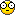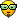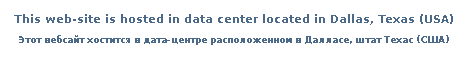Atom Feed | View unanswered posts | View active topics It is currently 09 May 2021 07:01Page 1 of 1 [ 11 posts ]
Great algorithm to convert an integer to ternary
Author Message
Maniac

Joined: 17 Sep 2012 14:36
Posts: 277
Location: 81.170.128.52This is fantastic. I've written an algorithm for host integer to trit array representation that is incredibly simple and fast. It's O(log(v)), all-integer and has no superfluous variables or overhead.Code:/* Convert a native integer v to * an array of at most n ternary digits, t. */void to_ternary(int* t, int n, int v) {        int i;         for(i = 0; i < n && v != 0; i++) {                if(v >= 0) {                        t[i] = v-3*((v+1)/3);                        v = (v+1)/3;                } else {                        t[i] = v-3*((v-1)/3);                        v = (v-1)/3;                }        }        for(; i < n; i++) t[i] = 0; }It's based on a mathematical insight I had on balanced bases when I was taking the bus earlier today. I wrote it down in a PDF if anyone is interested...

http://nedopc.org/ternary/tunguska/math ... .bases.pdf

The algorithm takes advantage of what happens to equation (8) when you let kappa be 1, and from that it's just a matter of inserting values into equation (11) and making optimizations.

22 Jan 2009 15:53Retired

Joined: 03 Aug 2003 23:37
Posts: 1477
Location: MoscowCongratulations! Very nice piece of quick working codeIt works with C# too (with small changes in definitions of course).

22 Jan 2009 16:20Maniac

Joined: 17 Sep 2012 14:36
Posts: 277
Location: 81.170.128.52Thanks. It should hopefully work with any language that where a*(b/a) is the same as a*floor(b/a) for integers a and b.

22 Jan 2009 16:26Hi,

I tried to understand...but could not,

i will try again23 Jan 2009 04:35Maniac

Joined: 17 Sep 2012 14:36
Posts: 277
Location: 81.170.128.52I find it's a very useful formulation. This function extracts an arbitrary digit in a host integer. It should be pretty easy to extend for systems that use wider words than 6.Code:/* Get the n:th trit in v */int tritn(int v, int n) {        if(v >= 0) {                switch(n) {                        case 0: return v - 3*((v+1)/3);                        case 1: return (v+1)/3 - 3*((v+4)/9);                        case 2: return (v+4)/9 - 3*((v+13)/27);                        case 3: return (v+13)/27 - 3*((v+40)/81);                        case 4: return (v+40)/81 - 3*((v+121)/243);                        case 5: return (v+121)/243 - 3*((v+364)/729);                                    }          } else {                switch(n) {                        case 0: return v + 3*((1-v)/3);                        case 1: return (v-1)/3 + 3*((4-v)/9);                        case 2: return (v-4)/9 + 3*((13-v)/27);                        case 3: return (v-13)/27 + 3*((40-v)/81);                        case 4: return (v-40)/81 + 3*((121-v)/243);                        case 5: return (v-121)/243 + 3*((364-v)/729);                }        }        return 0; }I haven't tested this one very extensively, but it seems to work. It allows you to set a trit in a host integer. It doesn't check so that t is in a valid range, so obviously care must be taken.Code:/* Set trit n in integer v to t */int settrit(int v, int n, int t) {        if(v >= 0) {                switch(n) {                        case 0:                                return t + 3*((v+1)/3);                        case 1:                                return v - 3*((v+1)/3 - t) + 9*((v+4)/9);                        case 2:                                return v - 9*((v+4)/9 - t) + 27*((v+13)/27);                        case 3:                                return v - 27*((v+13)/27 -t) + 81*((v+40)/81);                        case 4:                                return v - 81*((v+40)/81 -t) + 243*((v+121)/243);                        case 5:                                return v - 243*((v+121)/243 -t) + 729*((v+364)/729);                }        } else {                switch(n) {                        case 0:                                return t - 3*((1-v)/3);                        case 1:                                return v + 3*((1-v)/3 + t) + 9*((v-4)/9);                        case 2:                                return v + 9*((4-v)/9 + t) + 27*((v-13)/27);                        case 3:                                return v + 27*((13-v)/27 + t) + 81*((v-40)/81);                        case 4:                                return v + 81*((40-v)/81 + t) + 243*((v-121)/243);                        case 5:                                return v + 243*((121-v)/243 + t) + 729*((v-364)/729);                }        }        return 0;}23 Jan 2009 13:28Hi,

I am using the first code to convert int to Tryte....its useful....and i will be using 2nd one to perform memory operations in TVM...24 Jan 2009 02:07Maniac

Joined: 17 Sep 2012 14:36
Posts: 277
Location: 81.170.128.52Yeah, this method is incredibly useful. In Tunguska, I changed the linesCode:tryte instruction = memref(ip);tryte highbits = instruction >> 4;instruction = (instruction << 2) >> 2;toCode:int highbits, instruction = memref(ip).to_int();if(instruction > 0) {  highbits = ((instruction + 40) / 81);} else {  highbits = ((instruction-40) / 81);}instruction -= 81 * highbits;And got a 10-40% speed increase. I knew this was a bottleneck in the design, but yikes!On my system, it's now just below 3,000,000 operations per second!

24 Jan 2009 17:06Hi,
10-40% is a really good progress Waaw!!!!

Just wanted to know if you have developed any ternary algorithms for Matrix operations.

24 Jan 2009 18:57Maniac

Joined: 17 Sep 2012 14:36
Posts: 277
Location: 81.170.128.52Hmm, I'm not sure how that would be applicable. Most matrix operations are just a series of regular scalar operations. Base really doesn't factor in.

Though I have developed a wraparound function that allows you to use native integer arithmetic and still get "wraparound" of numbers bigger or smaller than what fits in the word.

For word size 6 (where of course 364=(3^6-1)/2 and 729=3^6):Code:int wrap(int v) {  if(v > 364) return v - (v+364)/729;  else if(v < -364) return v - (v-364)/729;  return v;}So then if you want to know something like a*b, you simply skip the intermediate trit array step by usingCode:int result = wrap(a*b);So you get like
wrap(364) = 364
wrap(365) = -364
wrap(366) = -363
wrap(-365) = 364
etc.

24 Jan 2009 19:24Thats an interesting function...i guess this can be used in case of representing Angels as they always lie between 0 t0 360 deg....so in ternary we can have them between "0-729"...yup its useful for matrix transform of 3D objects24 Jan 2009 19:45Joined: 09 Jan 2003 00:22
Posts: 19656
Location: Silicon Valley_________________https://twitter.com/Shaos1973

10 Nov 2012 09:55
Display posts from previous:  Sort byPage 1 of 1 [ 11 posts ]

#### Who is online

Users browsing this forum: Google [Bot] and 0 guests

 You cannot post new topics in this forumYou cannot reply to topics in this forumYou cannot edit your posts in this forumYou cannot delete your posts in this forumYou cannot post attachments in this forum

Search for:
 Jump to:  Select a forum ------------------ NEDOPC    Project nedoPC    Проект nedoPC TERNARY    Ternary (ENG)    Ternary (RUS) SPRINTER    Sprinter (ENG)    Sprinter (RUS) ZX SPECTRUM    ZX Spectrum (ENG)    ZX Spectrum (RUS) GENERAL    Hardware    Software    Emu80    Электронные штучки    Околонедописишности MICROS    INTEL    ZILOG    65XX    PIC    AVR    ARM    4-BIT    MCU/MPU RETRO    Radio-86RK    Orion-128/256    Spetsialist    PDP    MSX    IBM PC XT/AT    Soviet machines    Other retrocomputers ADMIN    Вебсайт и форумы